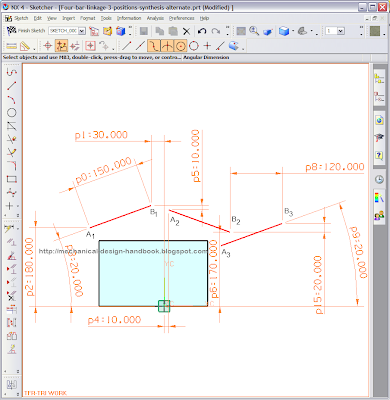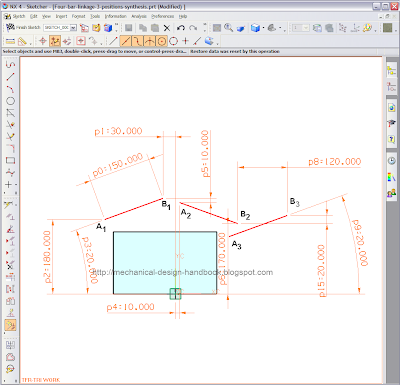## Posts

Showing posts from September, 2010

### Improve math skills of your kids - Learn step-by-step arithmetic from Math games

Math: Unknown - Step-by-step math calculation game for iOS.

Math: Unknown is much more than a math game. It is a step-by-step math calculation game which will teach users how to calculate in the correct order rather than just asking only the final calculated results.

The app consists of four basic arithmetic operations which are addition, subtraction, multiplication and division. In order to get started, users who are new to arithmetic can learn from animated calculation guides showing step-by-step procedures of solving each type of operation. It is also helpful for experienced users as a quick reference.

Generally, addition and subtraction may be difficult for users who just start learning math especially when questions require carrying or borrowing (also called regrouping). The app helps users to visualize the process of carrying and borrowing in the way it will be done on paper. Once users understand how these operations work, they are ready to learn multiplication and division.

For most students, division is considered as the most difficult arithmetic operation to solve. It is a common area of struggle since it requires prior knowledge of both multiplication and subtraction. To help users understand division, the app uses long division to teach all calculation procedures. Relevant multiplication table will be shown beside the question. Users will have to pick a number from the table which go into the dividend. Multiplication of selected number and divisor is automatically calculated, but the users have to do subtraction and drop down the next digit themselves. Learning whole calculation processes will make them master it in no time.

Math: Unknown is a helpful app for students who seriously want to improve arithmetic calculation skills.

### 3-Position Motion Generation Synthesis with Alternate Moving Pivots using Unigraphics NX4 SketchIn the previous post [3-Position Motion Generation Four-Bar Linkage Synthesis using Unigraphics NX4 Sketch], the locations of fixed pivots O2 and O4 are fixed due to the fixed locations of moving pivots A and B. Sometimes, the location of O2 and O4 are undesirable with respect to your design constraints. More flexible method to get desirable locations of O2 and O4 will be shown in this post.
1) Draw link AB in its three design positions A1B1, A2B2, and A3B3 as shown above.

2) Draw new attachment points C1 and D1 and other lines to form the rigid link ABDC. Do the same for position 2 and 3. Use "Constraint" command in Unigraphics NX4 sketch to set the equal length constraint to all relevant lines e.g. A1C1, A2C2, and A3C3 have the same length, but don't need to specify the fixed value for it. Once we complete these settings at all positions, it means we have set the fixed relationship between our desired line AB and other moving pivots CD. 3) Draw construction lines from …

### 3-Position Motion Generation Four-Bar Linkage Synthesis using Unigraphics NX4 SketchIn the real design problem, it will be more practical with 3 specified positions of a line in sequential order than 2-position synthesis. We can use a logical extension of the linkage synthesis technique described in [Four-bar linkage Synthesis using Unigraphics NX4 Sketch] to do the linkage synthesis with 3 positions.

Assume that we have to design the mechanism that move the link AB through 3 positions as shown below. The rectangle represents the limit of link AB, so link AB cannot interfere with the rectangle.

1) Draw link AB in its three design positions A1B1, A2B2, and A3B3 as shown above.
2) Draw construction lines from point A1 to A2 and A2 to A3

3) Bisect line A1A2 and line A2A3 and extend their perpendicular bisectors until they intersect with each other. Label the intersection O2.
4) Draw line O2A1. It's link 2.
5) Repeat the same for another end of the link. Draw construction lines from point B1 to B2 and B2 to B3

6) Bisect line B1B2 and line B2B3 and extend their perpen…

### Four-bar linkage Synthesis using Unigraphics NX4 SketchIn most design problems, we need to design the mechanism that moves between required positions. In this post, we will show the motion generation synthesis method to control a line in the plane to move in sequential positions. Any CAD software can be used to do this. But here we use Unigraphics NX4 sketch to draw and use "Animate Dimension" command to simulate the movement of all concerned links.

Assume that we need to design the mechanism to move line AB to A'B'. A simple and most commonly used four-bar linkage will be used here. Let's see how to find the fixed pivoting point at the ground.

1) Draw the link AB and A'B' in its desired positions in sketch of Unigraphics NX4 sketch (or any other CAD software) as shown in the above figure.

2) Draw construction lines from point A to A' and bisect line AA' and extend the perpendicular bisectors in convenience direction. (The choice is yours).

3) Select any convenient point on bisector as the fixed pivot…